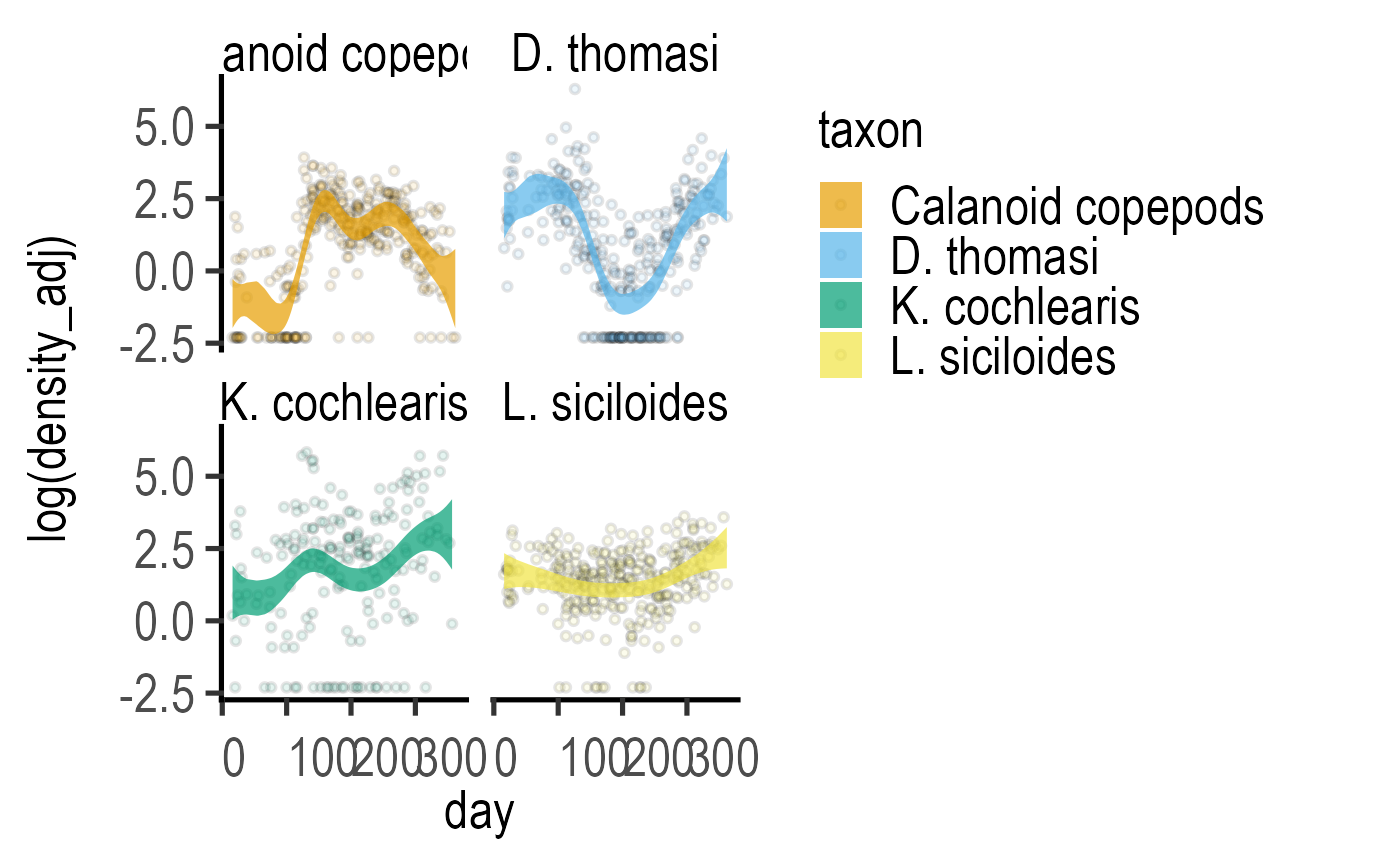Plot prediction of gam model

plot_gam_predict(
Model,
xcol,
ycol,
ByFactor,
symsize = 1,
s_alpha = 0.1,
smooth_alpha = 0.7,
linethick,
fontsize = 20,
...
)

## Arguments

Model

a generalised additive model (gam) fitted with ga_model or mgcv

xcol

the smooth in the gam (should match variable in the model exactly)

ycol

the dependent variable in gam (should match variable in the model exactly)

ByFactor

the by factor used in gam (should match variable in the model exactly)

symsize

size of symbols (default = 1)

s_alpha

opacity of symbols (default = 0.1)

smooth_alpha

opacity of the predicted CI interval (default = 0.7)

linethick

thickness of symbol lines (default = fontsize/22)

fontsize

base font size for graph

...

additional arguments to pass to plot_xy_CatGroup.

## Value

This function returns a ggplot2 object of class "gg" and "ggplot".

## Examples

#fit zooplankton data
z1 <- ga_model(data = data_zooplankton,
ycol = log(density_adj),